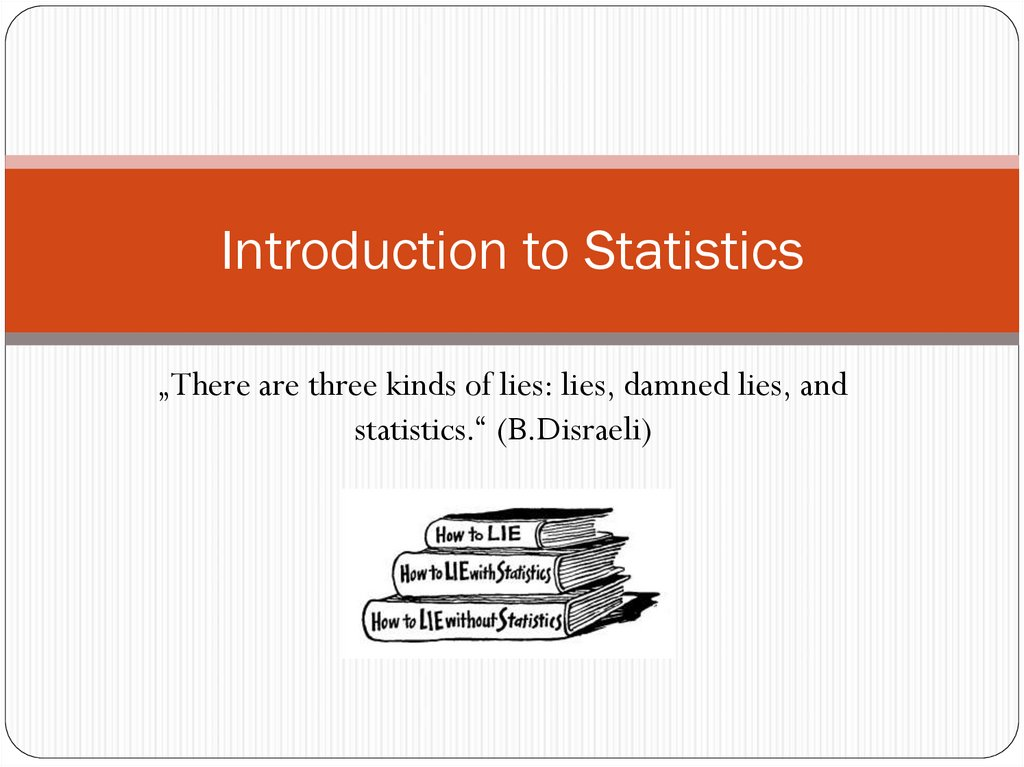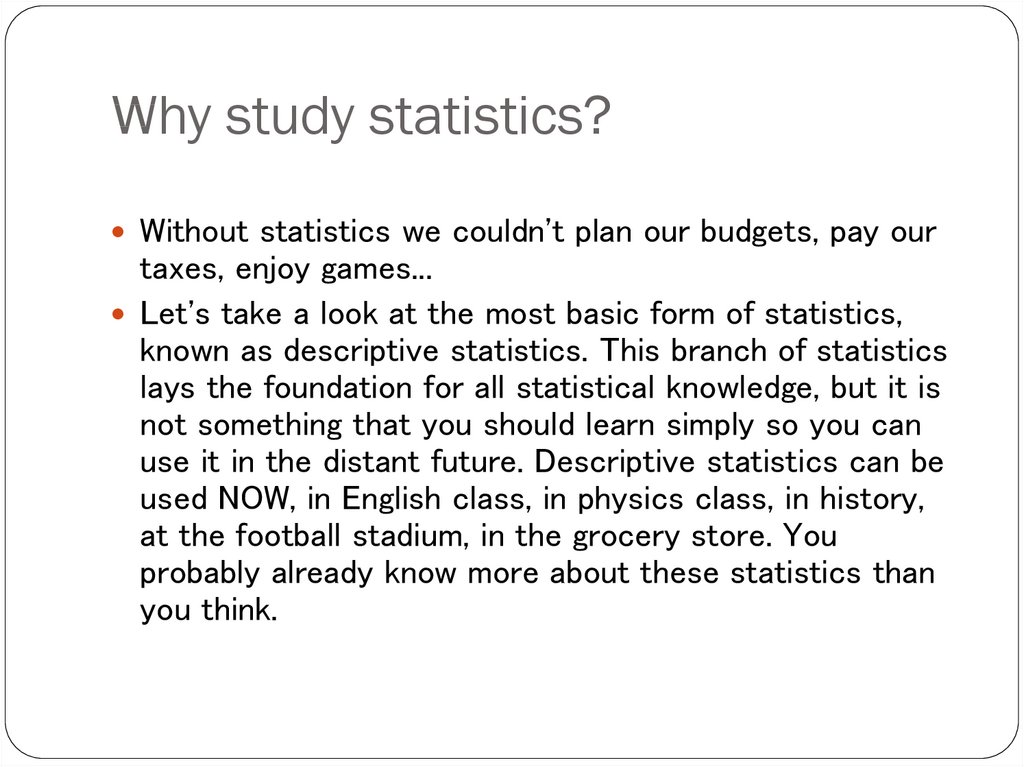# Introduction to Statistics

## 2. Introduction to Statistics

„There are three kinds of lies: lies, damned lies, and
statistics.“ (B.Disraeli)

## 3. Why study statistics?

Without statistics we couldn't plan our budgets, pay our
taxes, enjoy games...
Let's take a look at the most basic form of statistics,
known as descriptive statistics. This branch of statistics
lays the foundation for all statistical knowledge, but it is
not something that you should learn simply so you can
use it in the distant future. Descriptive statistics can be
used NOW, in English class, in physics class, in history,
at the football stadium, in the grocery store. You
you think.

## 4. Why study statistics?

Data are everywhere
2. Statistical techniques are used to make many decisions that
affect our lives
3. No matter what your career, you will make professional
decisions that involve data. An understanding of statistical
1.

## 5. Applications of statistical concepts in the business world

Finance – correlation and regression, index numbers, time
series analysis
Marketing – hypothesis testing, chi-square tests,
nonparametric statistics
Personel – hypothesis testing, chi-square tests,
nonparametric tests
Operating management – hypothesis testing, estimation,
analysis of variance, time series analysis

## 6. Statistics

The science of collecting, organizing, presenting, analyzing,
and interpreting data to assist in making more effective
decisions
Statistical analysis – used to manipulate summarize, and
investigate data, so that useful decision-making information
results.

## 7. Types of statistics

Descriptive statistics – Methods of organizing,
summarizing, and presenting data in an informative way
Inferential statistics – The methods used to determine
something about a population on the basis of a sample
Population –The entire set of individuals or objects of interest
or the measurements obtained from all individuals or objects of
interest
Sample – A portion, or part, of the population of interest

## 9. Inferential Statistics

Estimation
e.g., Estimate the population mean
weight using the sample mean weight
Hypothesis testing
e.g., Test the claim that the population
mean weight is 70 kg
Inference is the process of drawing conclusions or making decisions about a
population based on sample results

## 10. Sampling

a sample should have the same characteristics
as the population it is representing.
Sampling can be:
with replacement: a member of the population may be
chosen more than once (picking the candy from the bowl)
without replacement: a member of the population may
be chosen only once (lottery ticket)

## 11. Sampling methods

Sampling methods can be:
random (each member of the population has an equal chance of
being selected)
nonrandom
The actual process of sampling causes sampling
errors. For example, the sample may not be large
enough or representative of the population. Factors not
related to the sampling process cause nonsampling
errors. A defective counting device can cause a
nonsampling error.

## 12. Random sampling methods

simple random sample (each sample of the same size has an
equal chance of being selected)
stratified sample (divide the population into groups called
strata and then take a sample from each stratum)
cluster sample (divide the population into strata and then
randomly select some of the strata. All the members from these
strata are in the cluster sample.)
systematic sample (randomly select a starting point and take
every n-th piece of data from a listing of the population)

## 13. Descriptive Statistics

Collect data
e.g., Survey
Present data
e.g., Tables and graphs
Summarize data
e.g., Sample mean =
X
n
i

## 14. Statistical data

The collection of data that are relevant to the problem being
studied is commonly the most difficult, expensive, and timeconsuming part of the entire research project.
Statistical data are usually obtained by counting or measuring
items.
Primary data are collected specifically for the analysis desired
Secondary data have already been compiled and are available for
statistical analysis
A variable is an item of interest that can take on many different
numerical values.
A constant has a fixed numerical value.

## 15. Data

Statistical data are usually obtained by counting or measuring
items. Most data can be put into the following categories:
qualitative - data are measurements that each fail into one
of several categories. (hair color, ethnic groups and other
attributes of the population)
quantitative - data are observations that are measured on a
numerical scale (distance traveled to college, number of
children in a family, etc.)

## 16. Qualitative data

Qualitative data are generally described by words or
letters. They are not as widely used as quantitative data
because many numerical techniques do not apply to the
qualitative data. For example, it does not make sense to
find an average hair color or blood type.
Qualitative data can be separated into two subgroups:
dichotomic (if it takes the form of a word with two options
(gender - male or female)
polynomic (if it takes the form of a word with more than two
options (education - primary school, secondary school and
university).

## 17. Quantitative data

Quantitative data are always numbers and are the
result of counting or measuring attributes of a population.
Quantitative data can be separated into two
subgroups:
discrete (if it is the result of counting (the number of students of a
given ethnic group in a class, the number of books on a shelf, ...)
continuous (if it is the result of measuring (distance traveled,
weight of luggage, …)

## 18. Types of variables

Variables
Qualitative
Quantitative
Dichotomic
Polynomic
Discrete
Continuous
Gender, marital status
Brand of PC, hair
color
Children in family,
number of cars in a
parking lot
Amount of income tax
paid, weight of a
student

## 19. Numerical scale of measurement:

Nominal – consist of categories in each of which the number of respective
observations is recorded. The categories are in no logical order and have no
particular relationship. The categories are said to be mutually exclusive since an
individual, object, or measurement can be included in only one of them.
Ordinal – contain more information. Consists of distinct categories in which order
is implied. Values in one category are larger or smaller than values in other categories
(e.g. rating-excelent, good, fair, poor)
Interval – is a set of numerical measurements in which the distance between
numbers is of a known, constant size.
Ratio – consists of numerical measurements where the distance between numbers
is of a known, constant size, in addition, there is a nonarbitrary zero point.

## 20. Qualitative or Quantitative?

Preferred restaurant
Dollar amount of a loan
Height
Number of universities in Poland
Length of time to complete a task
Number of applicants
Ethnic origin

## 21.

Numerical presentation of qualitative
data
pivot table (qualitative dichotomic statistical attributes)
contingency table (qualitative statistical attributes from
which at least one of them is polynomic)
You should know how to convert absolute
values to relative ones (%).

## 22.

Frequency distributions – numerical
presentation of quantitative data
Frequency distribution – shows the frequency, or number of
occurences, in each of several categories. Frequency
distributions are used to summarize large volumes of data
values.
When the raw data are measured on a qunatitative scale,
either interval or ration, categories or classes must be
designed for the data values before a frequency distribution
can be formulated.

## 23. Steps for constructing a frequency distribution

1.
2.
3.
4.
5.
m n
Determine the number of classes
max min
Determine the size of each class
h
m
Determine the starting point for the first class
Tally the number of values that occur in each class
Prepare a table of the distribution using actual counts and/
or percentages (relative frequencies)

## 24. Frequency table

absolute frequency “ni” (Data Tab Data
Analysis Histogram)
relative frequency “fi”
Cumulative frequency distribution shows the total
number of occurrences that lie above or below certain key
values.
cumulative frequency “Ni”
cumulative relative frequency “Fi”

## 25. Charts and graphs

Frequency distributions are good ways to present the
essential aspects of data collections in concise and
understable terms
Pictures are always more effective in displaying large data
collections

## 26. Histogram

Frequently used to graphically present interval and ratio data
Is often used for interval and ratio data
The adjacent bars indicate that a numerical range is being
summarized by indicating the frequencies in arbitrarily
chosen classes

## 28. Frequency polygon

Another common method for graphically presenting interval
and ratio data
To construct a frequency polygon mark the frequencies on
the vertical axis and the values of the variable being measured
on the horizontal axis, as with the histogram.
If the purpose of presenting is comparation with other
distributions, the frequency polygon provides a good
summary of the data

## 30. Ogive

A graph of a cumulative frequency distribution
Ogive is used when one wants to determine how many
observations lie above or below a certain value in a
distribution.
First cumulative frequency distribution is constructed
Cumulative frequencies are plotted at the upper class limit of
each category
Ogive can also be constructed for a relative frequency
distribution.

## 32. Pie Chart

The pie chart is an effective way of displaying the percentage
breakdown of data by category.
Useful if the relative sizes of the data components are to be
emphasized
Pie charts also provide an effective way of presenting ratioor interval-scaled data after they have been organized into
categories

## 34. Bar chart

Another common method for graphically presenting nominal
and ordinal scaled data
One bar is used to represent the frequency for each category
The bars are usually positioned vertically with their bases
located on the horizontal axis of the graph
The bars are separated, and this is why such a graph is
frequently used for nominal and ordinal data – the separation
emphasize the plotting of frequencies for distinct categories

## 36. Time Series Graph

The time series graph is a graph of data that
have been measured over time.
The horizontal axis of this graph represents
time periods and the vertical axis shows the
numerical values corresponding to these
time periods